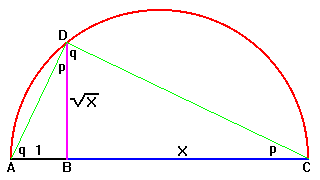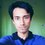# They're Not So Different!What we call mathematics is actually a really wide subject with a lot of branches, categories, sub-categories which are sometimes so different from one another that it's hard to believe that they are all coming from the same subject, mathematics. But when you start seeing the same thing from different perspectives, you'll see that somethings in mathematics, no matter how distant they seem, are actually equivalent. You'll see that different things are actually saying the same thing. To demonstrate that we'll see a visual proof of the AM-GM inequality for two variables. We'll learn that things aren't so different after all.

Before we get to the amazing proof of the AM-GM inequality, we'll need to learn a couple of things. First we're going to see how some geometrical constructions are equivalent to mathematical operations. We're going to learn to add, subtract, multiply, divide and take the square root of a number with a compass and a straightedge.Alt text

The image above demonstrates addition, subtraction, multiplication and division through geometric construction. Also pay attention to the colors. Addition and subtraction are pretty straightforward. Multiplication and division use the properties of similar figures. So we are able to perform the four basic arithmetic operations with a straightedge and compass. And these constructions aren't that difficult.

Taking the square root is a bit more complicated and it looks like this:Alt text

If you want to know why it works, here's the proof:

$ADC$ is a right angled triangle because all triangles inscribed in a semi-circle are right angled. A little bit of angle-chasing [and the picture shows how to do that] reveals to us that triangles $ABD$ and $DBC$ are similar. Thus we have,

$\frac{BD}{AB}=\frac{BC}{BD}$

Or, $BD^2=AB\times BC=x$

So, $BD=\sqrt{x}$.

Now that we've equipped ourselves with the knowledge required to prove the two variable case of the AM-GM inequality, let's get on with it.

The two variable case of the Arithmetic mean-Geometric mean inequality tells us given two positive numbers that are not equal, their arithmetic mean is always greater than their geometric mean. In other words, if $a$ and $b$ are two unequal positive numbers, then $\frac{a+b}{2}>\sqrt{ab}$.

If you've understood everything up to this point, this proof's going to be really easy for you. I'm not going to use any words for this! Behold:Alt text

The best part about this proof is that you can actually see that $\sqrt{ab}$ is smaller than $\frac{a+b}{2}$. You really don't need words for this [not entirely true, but the words needed were discussed earlier, so you know what I'm saying].

This was just an example [which is one of my favorites] to demonstrate that you could use different ways to say the same thing.

Note that I said nothing about taking the cubic root or fourth root or higher roots. This is because you can't do that with a straightedge and a compass. That is why you can't trisect an arbitrary angle or double a cube. But we'll discuss about this later someday.

Sources: The first two pictures were found through a Google search and then shamelessly stolen and modified. :)Note by Mursalin Habib
6 years, 10 months ago

This discussion board is a place to discuss our Daily Challenges and the math and science related to those challenges. Explanations are more than just a solution — they should explain the steps and thinking strategies that you used to obtain the solution. Comments should further the discussion of math and science.

When posting on Brilliant:

• Use the emojis to react to an explanation, whether you're congratulating a job well done , or just really confused .
• Ask specific questions about the challenge or the steps in somebody's explanation. Well-posed questions can add a lot to the discussion, but posting "I don't understand!" doesn't help anyone.
• Try to contribute something new to the discussion, whether it is an extension, generalization or other idea related to the challenge.

MarkdownAppears as
*italics* or _italics_ italics
**bold** or __bold__ bold
- bulleted- list
• bulleted
• list
1. numbered2. list
1. numbered
2. list
Note: you must add a full line of space before and after lists for them to show up correctly
paragraph 1paragraph 2

paragraph 1

paragraph 2

[example link](https://brilliant.org)example link
> This is a quote
This is a quote
    # I indented these lines
# 4 spaces, and now they show
# up as a code block.

print "hello world"
# I indented these lines
# 4 spaces, and now they show
# up as a code block.

print "hello world"
MathAppears as
Remember to wrap math in $$ ... $$ or $ ... $ to ensure proper formatting.
2 \times 3 $2 \times 3$
2^{34} $2^{34}$
a_{i-1} $a_{i-1}$
\frac{2}{3} $\frac{2}{3}$
\sqrt{2} $\sqrt{2}$
\sum_{i=1}^3 $\sum_{i=1}^3$
\sin \theta $\sin \theta$
\boxed{123} $\boxed{123}$

Sort by:

We need a tag of #ProofWithoutWords!

Staff - 6 years, 10 months ago

Impressive!!! The image in the proof also follows that when $a=b$, then obviously $BD$ and $EH$ are the same segment and so $BD=EH ~~~ \Longrightarrow ~~ \dfrac{a+b}{2}=\sqrt{ab}$.

- 6 years, 10 months ago

By drawing a suitable diagram, show that

$\sqrt{ \frac{a^2+b^2} { 2} } \geq \frac{ a+b}{2} \geq \sqrt{ab} \geq \frac{ ab}{a+b}.$

Staff - 6 years, 10 months ago

I think I can do that. Do you want me to post a solution or do you want others to work on it?

- 6 years, 10 months ago

The last expression in Calvin Sir's inequality I think should be $\frac{2ab}{a+b}$ and not $\frac{ab}{a+b}$,as all the other expressions mean Q.M,A.M,G.M and so I think the last one should be H.M.

- 6 years, 10 months ago

Note that $\frac{ab}{a+b} < \frac{2ab}{a+b}$ for positive numbers $a$ and $b$. So the inequality is still correct.

- 6 years, 10 months ago

Yeah. But I think he meant H.M only. Also, I think it will be hard to make a diagram with $\frac{ab}{a+b}$. P.S: I may be wrong.

- 6 years, 10 months ago

When you show that QM $\geq$ AM $\geq$ GM $\geq$ HM, it immediately follows that QM $\geq$ AM $\geq$ GM $\geq$ HM/2.

But you're probably right. He probably meant $\frac{2ab}{a+b}$ instead of $\frac{ab}{a+b}$.

- 6 years, 10 months ago

Oh sorry. I missed. Just bisecting the length H.Mwould give us the one we need.

- 6 years, 10 months ago

Yes, that is what I meant.

- 6 years, 10 months ago

Here's how to do it then. See this.

- 6 years, 10 months ago

Nice!

It turns out that the same sort of thing can bridge mathematics and computer science:

https://en.wikipedia.org/wiki/Curry%E2%80%93Howard_correspondence

However it's much less intuitive, it's a bit too complex for me to fully understand. But, the basic idea is that you can use computer programs to construct mathematical proofs. I won't go into it too much detail since this isn't a CS thread and I don't want to derail the discussion, but the idea is basically that, given a language with the correct sort of (very strict) restrictions, if you make certain assertions in your program, and you can get it to compile, you've made a proof.

Staff - 6 years, 10 months ago

Very well written; however, it seems that you got a little sidetracked. Only after I looked at the title after reading the article did I realize that it was about similarity between different fields of Mathematics, not about constructions. It would be more suitable, in my opinion, to have the name be Constructions and an example to be the proof of AM-GM.

- 6 years, 10 months ago

The similarity between the different fields of mathematics is a really big subject. I wanted to take a really small part of it and let people appreciate its beauty. I wanted to show people that you could view something in a completely different perspective. Yes, I agree that this post is a little constructions-heavy. But that is exactly what I wanted to do.

As always, your feedback is appreciated!

- 6 years, 10 months ago

It can also be proven using the MVT in Calculus. Nice!

- 6 years, 8 months ago